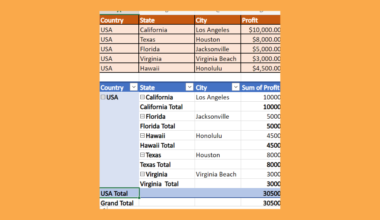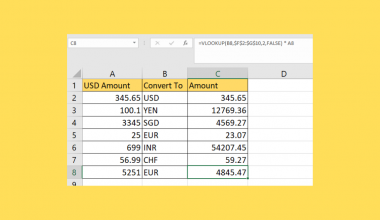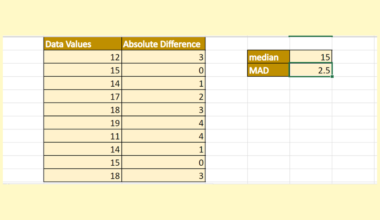# How to Use IF Statement Between Two Numbers or Dates

This guide will explain how to use the IF statement between two numbers or dates using the `IF` function and the `AND` function.

Excel is an excellent and powerful tool for many purposes. And one of the most common and used functions in Excel is the `IF` function. So the `IF` function will check whether a given condition is met. Then, it will return a value of TRUE or another value of FALSE, depending on whether the condition was met.

It allows us to compare data and make decisions based on the results. And the best part is we can combine the `IF` function with other functions to make it more dynamic.

For example, we can combine the `IF` function with the `AND` function to perform calculations between two different numbers or dates. And this can be very useful in many situations, especially in conditional situations.

Let’s take a sample scenario wherein we need to use the IF statement between two numbers or dates in Excel.

Suppose you are given a data set containing two values you must compare. After comparing the two numbers, you must return a TRUE or FALSE result. Since we have to use two numbers, we cannot simply use the `IF` function.

So we will be utilizing both the `IF` function and `AND` function to perform the task. In this case, it will check the conditions of both numbers and make a decision based on the two numbers.

Great! Before we move on, let’s first learn how to write the `IF` function in Excel.

## The Anatomy of the IF Function

The syntax or the way we write the `IF` function is as follows:

`=IF(logical_test, [value_if_true], [value_if_false])`

Let’s take apart this formula and understand what each term means:

• = the equal sign is how we begin any function in Excel.
• IF() is our `IF` function. And this function will check whether the given condition is met. Then, it will return a TRUE or FALSE depending on whether the condition was met or not.
• logical_test is a required argument. And it refers to any value or expression which can be evaluated to be TRUE or FALSE.
• value_if_true is an optional argument. And this refers to the value we want to be returned if the logical_test is true. By default, the function will return TRUE.
• value_if_false is another optional argument. Similarly, it refers to any value we want to be returned if the logical_test is false. By default, the function will return FALSE.

Amazing! Now let’s learn how to write the `AND` function in Excel.

## The Anatomy of the AND Function

The syntax or the way we write the `AND` function is as follows:

`=AND(logical1, [logical2])`

Let’s take apart this formula and understand what each term means:

• = the equal sign is how we start any function in Excel.
• AND() is our `AND` function. And this function will check whether all arguments are TRUE. Then, it will return TRUE if all arguments are TRUE.
• logical1 is a required argument. And it refers to the condition we want to test that can either be TRUE or FALSE. So this can be arrays, logical values, or cell references.
• logical2 is an optional argument. And it refers to another condition we want to test. Also, this can be logical values, arrays, or cell references.

Finally, let’s dive into a real example of using the IF statement between two numbers or dates in Excel.

## A Real Example of Using IF Statement Between Two Numbers or Dates

Let’s say we want to compare two numbers in our data set. Then, we want to return a specific value depending on whether the results are TRUE or FALSE. In this case, our initial data set would look like this:So we can compare two numbers by combining the `IF` function and the `AND` function. Importantly, we will also be using comparison operators. So let’s learn about the different comparison operators we can use in our `IF` function.

Firstly, we have the equal to, symbolized by an equal sign, or =. Secondly, we have the condition not equal to, symbolized as <>. Thirdly, we have greater than with the symbol >.

Next, we have the condition lesser than with the operator <. Then, we have the condition greater than or equal to, symbolized by >=. Lastly, we have lesser than or equal to, symbolized by <=.

So we can make use of these 6 comparison operators to create our conditions within the `IF` function and `AND` function.

For example, we want to return a Yes if the value is between 50 and 100. And we want to return a No if the value is not between 50 and 100. So we simply combine the `IF` function and `AND` function and use the appropriate comparison operators within the functions.

Furthermore, we can also use the `IF` function and `AND` function between dates. Essentially, the process of using the function between numbers and between dates is similar. For instance, we want to check if a specified date is within a given range.

So the generic formula for this would be `=IF(AND(date>= start_date, date <= end_date, value_if_true, value_if_false)`. However, we should remember that we need to wrap the formula in the `DATEVALUE` function. Because the `IF` function does not recognize the date values as dates, it recognizes them as text strings.

Finally, our final output would be something like this:Great! Let’s move on and learn the steps of how to use IF statement between two numbers or dates.

## How to Use IF Statement Between Two Numbers or Dates

In this section, we will discuss the step-by-step process of how to use IF statement between two numbers or dates by combining the `IF` function and `AND` function in Excel.

1. Firstly, we will use the functions between two numbers. To do this, we can simply type in the formula “=IF(AND(B3>=50, B3<=100), “Yes”, “No”)”. Then, press the Enter key to return the results.2. Next, drag down to copy the formula below.3. And tada! We have successfully used the IF statement between two numbers.4. Secondly, let’s use the IF statement between two dates. To do this, input the formula “=IF(AND(E3>=DATEVALUE(“1/1/2022”),E3<=DATEVALUE(“12/1/2022”)), “Yes”, “No”)“. Finally, press the Enter key to return the results.5. Next, drag down to copy the formula.6. And tada! We have used the IF statement between two numbers or dates.And that’s pretty much it! We have discussed how to use the IF statement between two numbers or dates by combining the `IF` and `AND` functions. Now you can apply this whenever you need to.

Are you interested in learning more about what Excel can do? You can now use the `IF` function and the various other Microsoft Excel formulas available to create great worksheets that work for you. Make sure to subscribe to our newsletter to be the first to know about the latest guides and tutorials from us.Our goal this year is to create lots of rich, bite-sized tutorials for Google Sheets users like you. If you liked this one, you'll love what we are working on! Readers receive ✨ early access ✨ to new content.

##### You May Also Like## How to Create Multi Level Hierarchy in Excel

This guide will explain how to create a multi-level hierarchy in Excel using two easy and efficient methods. …## How to Calculate Percentage Difference in Excel

This guide will explain how to calculate the percentage difference in Excel. Similar to percentage change, the percentage…## How To Use NPER Function in Excel

The NPER function in Excel is useful when you need to find out the number of periods for…## How to Do Currency Conversion using VLOOKUP in Excel

This guide will explain how we can perform currency conversion using the VLOOKUP function in Excel. The VLOOKUP…